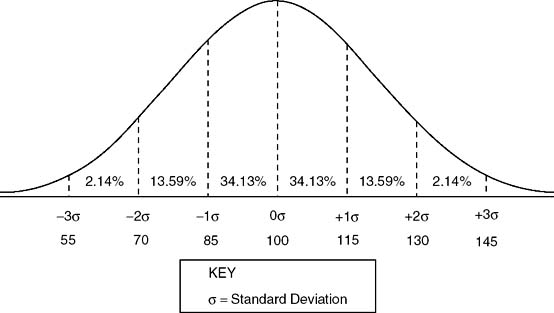# Normal Distribution

The normal distribution is a hypothetical distribution of individual scores on any test. The normal distribution is a symmetrically shaped bell curve with the largest number of scores in the middle of the distribution and the smallest number of scores in the tails of the distribution (Figure 1). Because the normal curve is symmetrical, the measures of central tendency (i.e., the mean, median, and mode) will be the same. The mode is the most frequent score in the distribution, the mean is the average score in the distribution, and the median is the middlemost score or the midpoint of the distribution (Cohen & Swerdlik, 1999).

### Figure 1 Normal CurveThe normal distribution can be “divided into areas defined in standard deviation units” (Cohen & Swerdlik, 1999, p. ...

•••